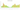# د.ك.‏

## Kuwaiti Dinar

Kuwait's official currency is the dinar. It has 1,000 fils as its subdivisions. With KD 1 equaling US\$3.32 in 2022, the Kuwaiti dinar will be the currency with the highest value per base unit, ahead of the Bahraini dinar with BD 1 equaling US\$2.65.

#### how has the value of the currency changed in the last year?## USD 1 = KWD 0.30911

How much is10 US dollars worth inKuwaiti dinars?
At the current exchange rate, 10 US dollars is worth 3.09 Kuwaiti dinars
How much is50 US dollars worth inKuwaiti dinars?
At the current exchange rate, 50 US dollars is worth 15.46 Kuwaiti dinars
How much is100 US dollars worth inKuwaiti dinars?
At the current exchange rate, 100 US dollars is worth 30.91 Kuwaiti dinars
How much is500 US dollars worth inKuwaiti dinars?
At the current exchange rate, 500 US dollars is worth 154.56 Kuwaiti dinars
How much is2,000 US dollars worth inKuwaiti dinars?
At the current exchange rate, 2,000 US dollars is worth 618.23 Kuwaiti dinars
How much is10 US dollars worth inKuwaiti dinars?
At the current exchange rate, 10 US dollars is worth 3.09 Kuwaiti dinars
How much is50 US dollars worth inKuwaiti dinars?
At the current exchange rate, 50 US dollars is worth 15.46 Kuwaiti dinars
How much is100 US dollars worth inKuwaiti dinars?
At the current exchange rate, 100 US dollars is worth 30.91 Kuwaiti dinars
How much is500 US dollars worth inKuwaiti dinars?
At the current exchange rate, 500 US dollars is worth 154.56 Kuwaiti dinars
How much is2,000 US dollars worth inKuwaiti dinars?
At the current exchange rate, 2,000 US dollars is worth 618.23 Kuwaiti dinars